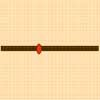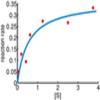# Search by Topic

#### Resources tagged with Turning points similar to Folium of Descartes:

Filter by: Content type:
Age range:
Challenge level:

### There are 11 results

Broad Topics > Calculus > Turning points### Folium of Descartes

##### Age 16 to 18 Challenge Level:

Investigate the family of graphs given by the equation x^3+y^3=3axy for different values of the constant a.### Witch of Agnesi

##### Age 16 to 18 Challenge Level:

Sketch the members of the family of graphs given by y = a^3/(x^2+a^2) for a=1, 2 and 3.### Curvy Catalogue

##### Age 16 to 18 Challenge Level:

Make a catalogue of curves with various properties.### Curve Fitter

##### Age 16 to 18 Challenge Level:

Can you fit a cubic equation to this graph?### Patterns of Inflection

##### Age 16 to 18 Challenge Level:

Find the relationship between the locations of points of inflection, maxima and minima of functions.### Scientific Curves

##### Age 16 to 18 Challenge Level:

Can you sketch these difficult curves, which have uses in mathematical modelling?### Quick Route

##### Age 16 to 18 Challenge Level:

What is the quickest route across a ploughed field when your speed around the edge is greater?### Calculus Analogies

##### Age 16 to 18 Challenge Level:

Consider these analogies for helping to understand key concepts in calculus.### Least of All

##### Age 16 to 18 Challenge Level:

A point moves on a line segment. A function depends on the position of the point. Where do you expect the point to be for a minimum of this function to occur.### Bird-brained

##### Age 16 to 18 Challenge Level:

How many eggs should a bird lay to maximise the number of chicks that will hatch? An introduction to optimisation.### Curve Fitter 2

##### Age 16 to 18 Challenge Level:

Can you construct a cubic equation with a certain distance between its turning points?